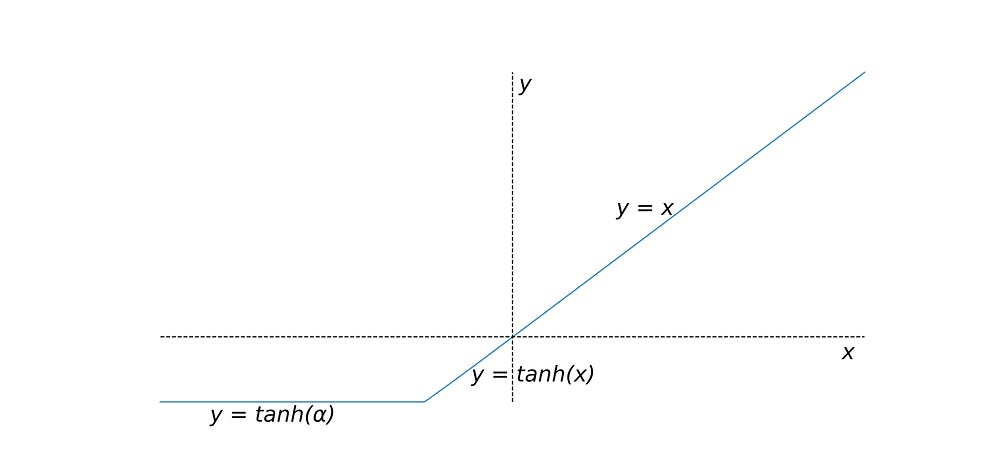# A New Hyperbolic Tangent Based Activation Function for Neural Networks

Source: Deep Learning on Medium

A New Hyperbolic Tangent Based Activation Function for Neural Networks

Abstract:

This article introduces a new hyperbolic tangent based activation function, tangent linear unit (TaLU), for neural networks. The function was evaluated for performance using CIFAR-10 and CIFAR-100 database. The performance of the proposed activation function was in par or better than other activation functions such as: standard rectified linear unit (ReLU), leaky rectified linear unit (Leaky ReLU), and exponential linear unit (ELU).

Introduction:

The rectified linear unit (ReLU)(Nair V. and Hinton, G. E., “Rectified linear units improve restricted Boltzmann machines”, ICML, 2010, pp. 807–810) is one of the most popular non-saturated activation functions, used in neural networks. However, ReLU suffers from the problem of dying ReLU, where some of the neurons starts to output 0. Sometimes, half the neurons die, in particular if they are used with large learning rate (Géron, A., “Hands-On Machine Learning with Scikit-Learn & TensorFlow”, 1st ed., O’Reilly Media, Inc., 2017, p. 281). To circumvent these problems, Xu et al. ( Xu, B., Wang, N., Chen, T., Li, M., “Emperical Evaluation of Rectified Activations in Convolution Network”, aerXiv preprint arXiv:1505.00853v2, 2015)evaluated variants of ReLU, such as leaky rectified linear unit (leaky ReLU), parametric rectified linear unit (PReLU) and randomized rectified linear unit (RReLU). It was observed that leaky ReLU mostly outperformed the standard ReLU. Clevert et al. (Clevert, D., Unterthiner, T., Hochreiter, S., “Fast and Accurate Deep Network Learning by Exponential Linear Units”, aerXiv preprint arXiv:1511.07289, 2015) proposed exponential linear unit (ELU) that was observed to outperform all the variants of ReLU (Géron, A., “Hands-On Machine Learning with Scikit-Learn & TensorFlow”, 1st ed., O’Reilly Media, Inc., 2017, p. 282).

This paper proposes a new activation function based on hyperbolic tangent function, tangent linear unit (TaLU). Discussed below are the definition of TaLU, followed by determination of its optimized parameters, followed by its comparison with other activation functions such as ReLU, leaky ReLU and ELU.

Tangent Linear Unit (TaLU):

The tangent linear unit (TaLu) can be illustrated in Figure 1, and can be described by equation below.

Figure 1: Tangent Linear Unit (TaLU)

where α is a fixed parameter with values < 0. α was tested from -0.50 to -0.01 in this article.

Experimental Setup:

The proposed activation function was tested on CIFAR-10 and CIFAR-100 datasets, and the performance was compared with ReLU, leaky ReLu (α = 0.01) and ELU (α = 1). The python-based libraries: Tensorflow, Numpy, Pandas and Keras library were used during this study. The neural network architecture is described in Figure 2. The data was divided into train and validation in the ratio of 9:1 from the train datasets provided for CIFAR-10 and CIFAR-100, and the model was tested on test datasets provided for CIFAR-10 and CIFAR-100.

Figure 2: The architecture of the neural network used during this study (Note: the activation function (af) in Layers 1, 2, 5, 7, 11 was Talu, ReLU, ELU, and leaky ReLU, based on the experiment).

The code can be found at https://github.com/mjain72.

Parametric Study for TaLU:

Initially, a parametric study was performed to determine the optimum value of α. Table 1 shows the loss and accuracy values for train, validation and test for CIFAR-10 dataset. The number of epochs were 25, during training. The optimum value of α was found to be -0.05. Similar optimal value for α was observed for CIFAR-100 dataset (Table 2).

Table 1: Parametric Study for TaLU using CIFAR-10 dataset

Table 2: Parametric Study for TaLU using CIFAR-100 dataset

Based on these studies, it was decided to use α = -0.05 for TaLU, during the comparison study with ReLU, leaky ReLU and ELU activation functions.

Comparative study of TaLU with various activation functions:

Once it was determined that the optimum value of α is -0.05, in case of TaLU, we used this value to perform a comparative study, with other activation functions. Table 3 shows the performance of various functions, in the case of CIFAR-100 dataset. The performance of TaLU was observed to be superior, in terms of accuracy, compared to other activation functions.

Table 3: Performance of various activation functions for CIFAR-100 dataset

Table 4, shows the performance of various activation functions, in the case of CIFAR-10 dataset. In this case also, the performance of TaLU was in par or superior to other activation functions.

Table 4: Performance of various activation functions for CIFAR-10 dataset

Conclusions:

Based on the above study, it can be concluded that the proposed activation function, TaLU, provides a better or similar performance as currently used activation functions, such as ReLU, leaky ReLU and ELU, and should be evaluated for future studies.

Convergence Curves:

Figure 3: Convergence curves for TaLU at different α values, shown in parenthesis, using CIFAR-10 dataset.

Figure 4: Convergence curves for TaLU at different α values, shown in parenthesis, using CIFAR-100 dataset.

Figure 5: Convergence curves for different activation functions, using CIFAR-10 dataset.

Figure 6: Convergence curves, for different activation functions, for CIFAR-100 dataset.